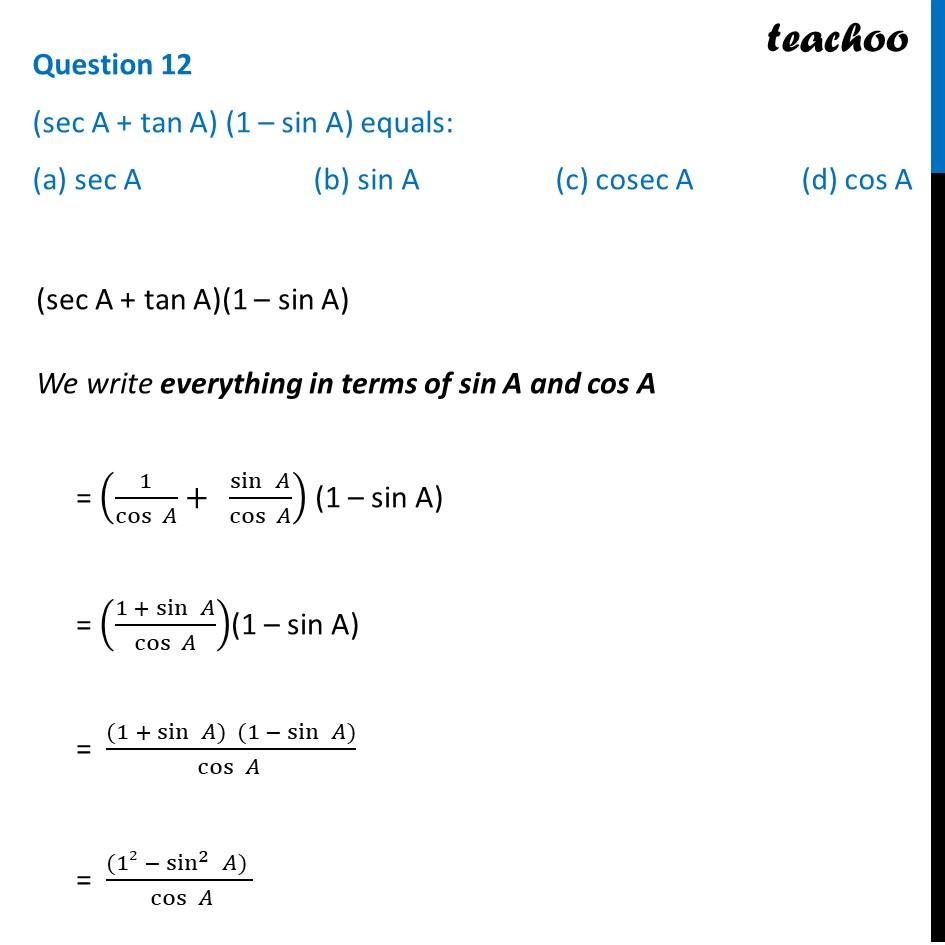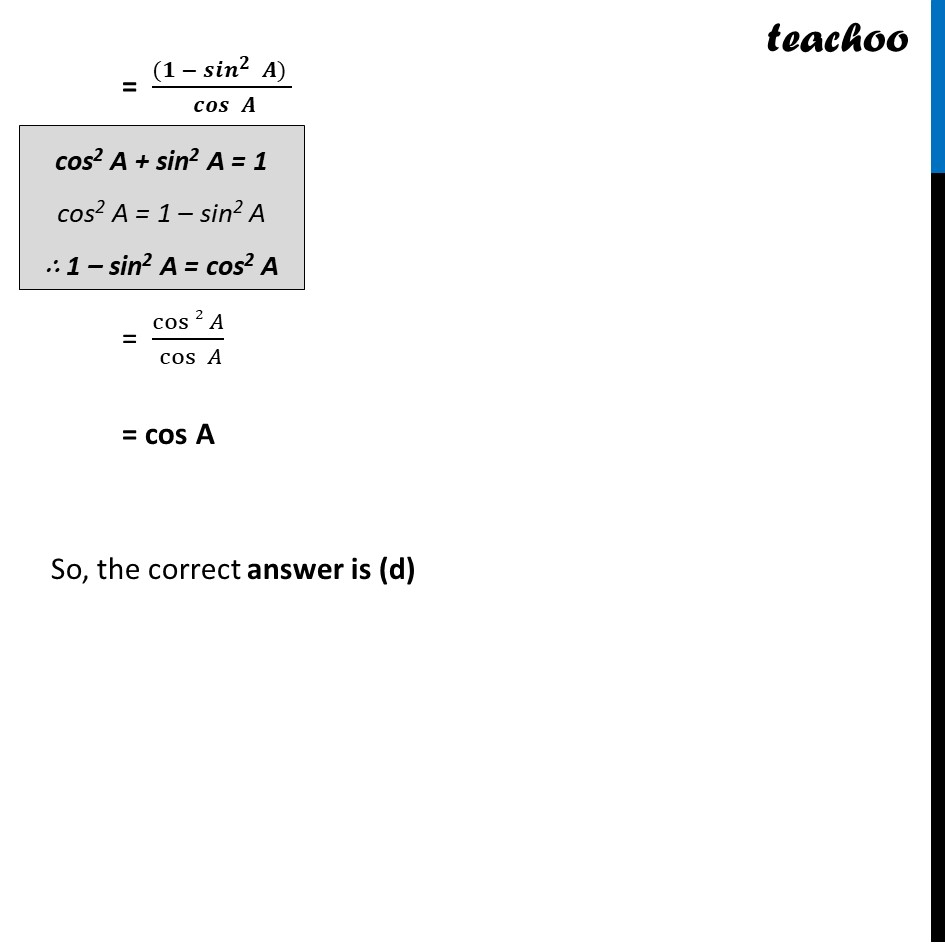CBSE Class 10 Sample Paper for 2024 Boards - Maths Standard

Class 10
Solutions of Sample Papers for Class 10 Boards

## (a) sec A                        (b) sin A                   (c) cosec A               (d) cos A

This question is similar to Example 10 - Chapter 8 Class 10 Introduction to TrignometryLearn in your speed, with individual attention - Teachoo Maths 1-on-1 Class

### Transcript

(sec A + tan A)(1 – sin A) We write everything in terms of sin A and cos A = (1/cos⁡〖 𝐴〗 +" " sin⁡〖 𝐴〗/cos⁡〖 𝐴〗 ) (1 – sin A) = ((1 +〖 sin〗⁡〖 𝐴〗)/cos⁡〖 𝐴〗 )(1 – sin A) = ((1 +〖 sin〗⁡〖 𝐴) (1 −〖 sin〗⁡〖 𝐴)〗 〗)/(cos⁡ 𝐴 ) = ((12 −〖 sin^2〗⁡〖 𝐴) 〗)/( cos⁡〖 𝐴〗 ) = ((𝟏 −〖 〖𝒔𝒊𝒏〗^𝟐〗⁡〖 𝑨) 〗)/( 𝒄𝒐𝒔⁡〖 𝑨〗 ) = cos⁡〖2 𝐴〗/( cos⁡〖 𝐴〗 ) = cos A cos2 A + sin2 A = 1 cos2 A = 1 – sin2 A ∴ 1 – sin2 A = cos2 A So, the correct answer is (d)